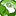# New to Qlik Sense

If you’re new to Qlik Sense, start with this Discussion Board and get up-to-speed quickly.

Announcements
Qlik Insider - Maximize your investment, Join this webinar, May 26th. Register Today
cancel
Showing results for
Did you mean:Creator

## If statement with set analysis

I am trying with a variable and If statement to render a bubble graph based on selections. I get all to work except the below syntax:

=if(\$(vSelect)=1,(sum(if(Year2Date(PERIOD,0,if(MONTH(LatestDate)>11,1,MONTH(LatestDate)+1),LatestDate),\$(vMeasure)))/
(sum(TOTAL(if(Year2Date(PERIOD,0,if(MONTH(LatestDate)>11,1,MONTH(LatestDate)+1),LatestDate),\$(vMeasure))))))),

if(\$(vSelect)=2,(SUM(IF(Year2Date(PERIOD,0,1,LatestDate),\$(vMeasure)))/SUM(TOTAL(IF(Year2Date(PERIOD,0,1,LatestDate),\$(vMeasure))))))

What am I missing ?

Regards

1 Solution

Accepted SolutionsMVP

Try this

=if(\$(vSelect)=1,(sum(if(Year2Date(PERIOD,0,if(MONTH(LatestDate)>11,1,MONTH(LatestDate)+1),LatestDate),\$(vMeasure)))/
(sum(TOTAL(if(Year2Date(PERIOD,0,if(MONTH(LatestDate)>11,1,MONTH(LatestDate)+1),LatestDate),\$(vMeasure)))))),

if(\$(vSelect)=2,(SUM(IF(Year2Date(PERIOD,0,1,LatestDate),\$(vMeasure)))/SUM(TOTAL(IF(Year2Date(PERIOD,0,1,LatestDate),\$(vMeasure)))))))

Nested if should be like this

= If( Condition1, Exp1 , If(Condition1, Exp2, Exp3(default)))MVP

Try this

=if(\$(vSelect)=1,(sum(if(Year2Date(PERIOD,0,if(MONTH(LatestDate)>11,1,MONTH(LatestDate)+1),LatestDate),\$(vMeasure)))/
(sum(TOTAL(if(Year2Date(PERIOD,0,if(MONTH(LatestDate)>11,1,MONTH(LatestDate)+1),LatestDate),\$(vMeasure)))))),

if(\$(vSelect)=2,(SUM(IF(Year2Date(PERIOD,0,1,LatestDate),\$(vMeasure)))/SUM(TOTAL(IF(Year2Date(PERIOD,0,1,LatestDate),\$(vMeasure)))))))

Nested if should be like this

= If( Condition1, Exp1 , If(Condition1, Exp2, Exp3(default)))Tags